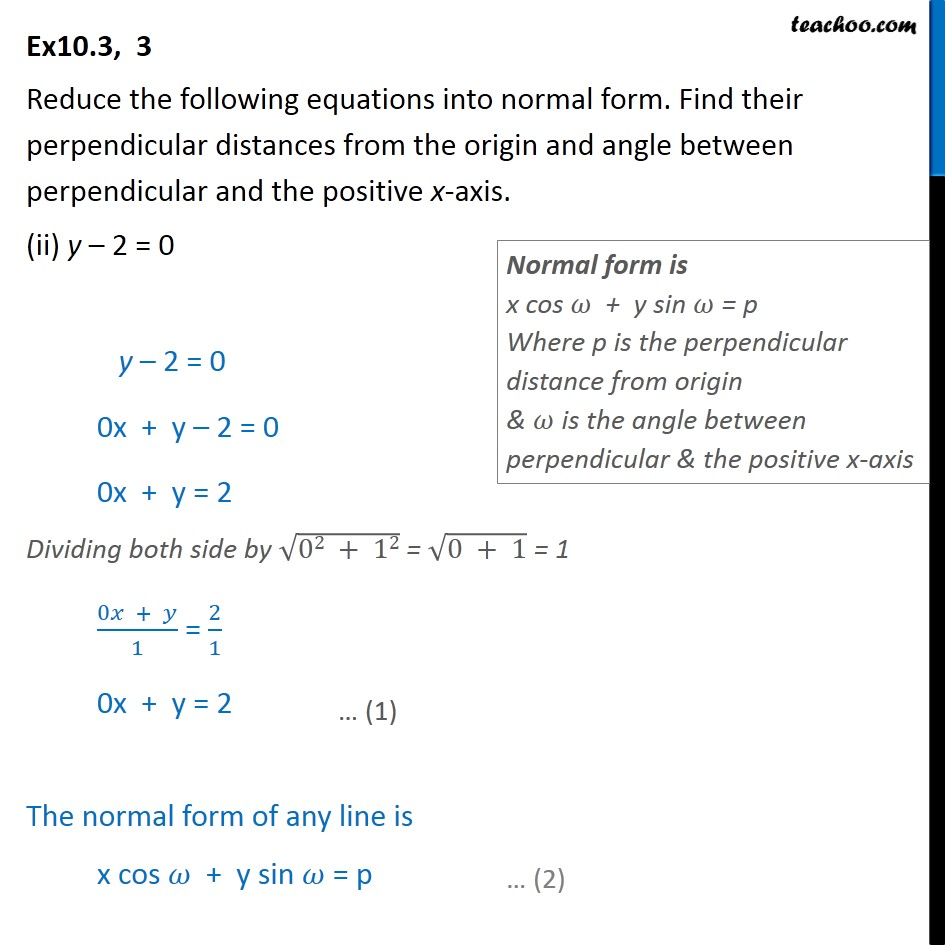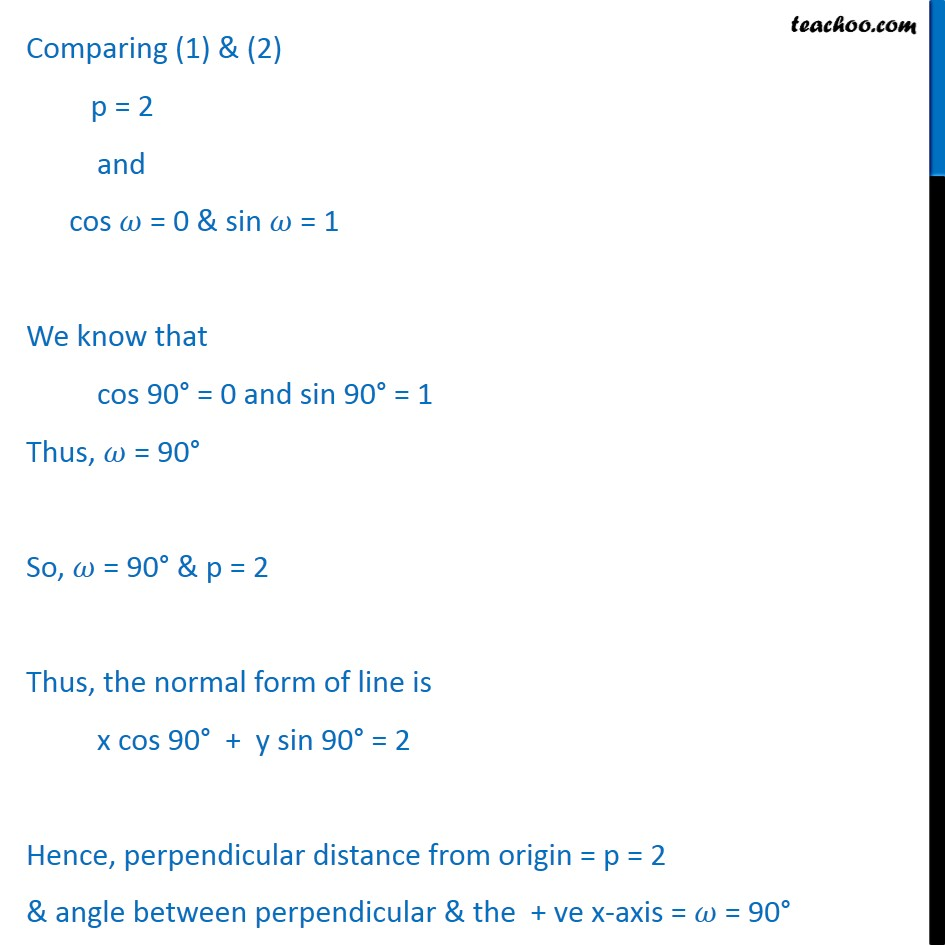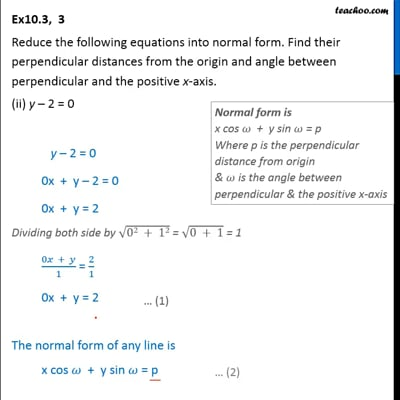Ex 10.3

Chapter 10 Class 11 Straight Lines
Serial order wiseThis video is only available for Teachoo black users

Maths Crash Course - Live lectures + all videos + Real time Doubt solving!

### Transcript

Ex10.3, 3 Reduce the following equations into normal form. Find their perpendicular distances from the origin and angle between perpendicular and the positive x-axis. (ii) y 2 = 0 y 2 = 0 0x + y 2 = 0 0x + y = 2 Dividing both side by (02 + 12) = (0 + 1) = 1 (0 + )/1 = 2/1 0x + y = 2 The normal form of any line is x cos + y sin = p Comparing (1) & (2) p = 2 and cos = 0 & sin = 1 We know that cos 90 = 0 and sin 90 = 1 Thus, = 90 So, = 90 & p = 2 Thus, the normal form of line is x cos 90 + y sin 90 = 2 Hence, perpendicular distance from origin = p = 2 & angle between perpendicular & the + ve x-axis = = 90